The CUSUM Procedure

### Creating a One-Sided Cusum Chart with a Decision Interval

[See CUSONES1 in the SAS/QC Sample Library]An alternative to the V-mask cusum chart is the one-sided cusum chart with a decision interval, which is sometimes referred to as the "computational form of the cusum chart." This example illustrates how you can create a one-sided cusum chart for individual measurements.

A can of oil is selected every hour for fifteen hours. The cans are weighed, and their weights are saved in a SAS data set named Cans:1

```data Cans;
length comment \$16;
label Hour = 'Hour';
input Hour Weight comment \$16. ;
datalines;
1  8.024
2  7.971
3  8.125
4  8.123
5  8.068
8  8.072
9  8.066
10  8.089
11  8.058
12  8.147
13  8.141
14  8.047
15  8.125
;
run;
```

Suppose the problem is to detect a positive shift in the process mean of one standard deviation (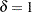) from the target of 8.100 ounces. Furthermore, suppose that

• a known value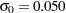is available for the process standard deviation

• an in-control average run length (ARL) of approximately 100 is required

• an ARL of approximately five is appropriate for detecting the shift

Table 6.3 indicates that these ARLs can be achieved with the decision interval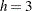and the reference value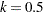. The following statements use these parameters to create the chart and tabulate the cusum scheme:

```options nogstyle;
goptions ftext=swiss;
symbol v=dot color=salmon h=1.8 pct;
title "One-Sided Cusum Analysis";
proc cusum data=Cans;
xchart Weight*Hour /
mu0      = 8.100     /* target mean for process     */
sigma0   = 0.050     /* known standard deviation    */
delta    = 1         /* shift to be detected        */
h        = 3         /* cusum parameter h           */
k        = 0.5       /* cusum parameter k           */
scheme   = onesided  /* one-sided decision interval */
tableall             /* table                       */
cinfill  = ywh
cframe   = bigb
cout     = salmon
cconnect = salmon
climits  = black
coutfill = bilg;
label Weight = 'Cusum of Weight';
run;
options gstyle;
```

The NOGSTYLE system option causes ODS styles not to affect traditional graphics. Instead, the SYMBOL statement, GOPTIONS, and XCHART statement options control the appearance of the graph. The GSTYLE system option restores the use of ODS styles for traditional graphics produced subsequently. The chart is shown in Figure 6.6.

Figure 6.6 One-Sided Cusum Chart with Decision Interval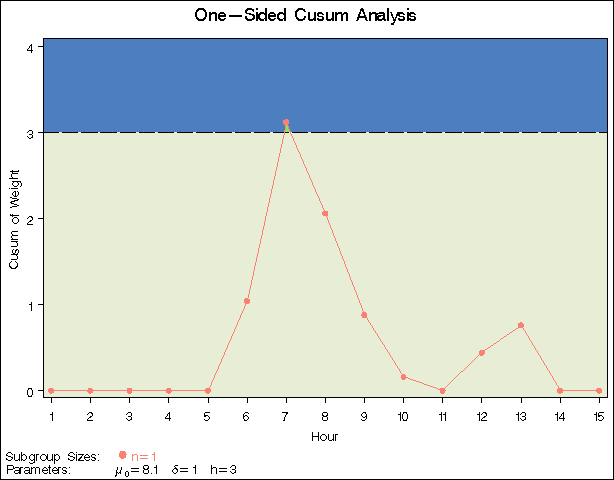The cusum plotted at Hour=t is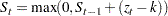where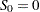, and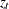is the standardized deviation of theth measurement from the target.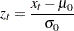The cusum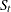is referred to as an upper cumulative sum. A shift is signaled at the seventh hour since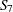exceeds. For further details, see One-Sided Cusum Schemes.

The option TABLEALL requests the tables shown in Figure 6.7, Figure 6.8, and Figure 6.9. The table in Figure 6.7 summarizes the cusum scheme, and it confirms that an in-control ARL of 117.6 and an ARL of 6.4 atare achieved with the specifiedand.

Figure 6.7 Summary Table
Cusum Parameters
Process Variable Weight (Cusum of Weight)
Subgroup Variable Hour (Hour)
Scheme One-Sided
Target Mean (Mu0) 8.1
Sigma0 0.05
Delta 1
Nominal Sample Size 1
h 3
k 0.5
Average Run Length (Delta) 6.40390895
Average Run Length (0) 117.595692

The table in Figure 6.8 tabulates the information displayed in Figure 6.6.

Figure 6.8 Tabulation of One-Sided Chart
 One-Sided Cusum Analysis

The CUSUM Procedure

Cumulative Sum Chart Summary for Weight
Hour Subgroup
Sample
Size
Individual
Value
Cusum Decision
Interval
Decision
Interval
Exceeded
1 1 8.0240000 0.0000000 3.0000
2 1 7.9710000 0.0000000 3.0000
3 1 8.1250000 0.0000000 3.0000
4 1 8.1230000 0.0000000 3.0000
5 1 8.0680000 0.0000000 3.0000
6 1 8.1770000 1.0400000 3.0000
7 1 8.2290000 3.1200000 3.0000 Upper
8 1 8.0720000 2.0600000 3.0000
9 1 8.0660000 0.8800000 3.0000
10 1 8.0890000 0.1600000 3.0000
11 1 8.0580000 0.0000000 3.0000
12 1 8.1470000 0.4400000 3.0000
13 1 8.1410000 0.7600000 3.0000
14 1 8.0470000 0.0000000 3.0000
15 1 8.1250000 0.0000000 3.0000

The table in Figure 6.9 presents the computational form of the cusum scheme described by Lucas (1976).

Figure 6.9 Computational Form of Cusum Scheme
 One-Sided Cusum Analysis

The CUSUM Procedure

Computational Cumulative Sum for Weight
Hour Subgroup
Sample
Size
Individual
Value
Upper
Cusum
Number of
Consecutive
Upper Sums > 0
1 1 8.0240000 0.0000000 0
2 1 7.9710000 0.0000000 0
3 1 8.1250000 0.0000000 0
4 1 8.1230000 0.0000000 0
5 1 8.0680000 0.0000000 0
6 1 8.1770000 1.0400000 1
7 1 8.2290000 3.1200000 2
8 1 8.0720000 2.0600000 3
9 1 8.0660000 0.8800000 4
10 1 8.0890000 0.1600000 5
11 1 8.0580000 0.0000000 0
12 1 8.1470000 0.4400000 1
13 1 8.1410000 0.7600000 2
14 1 8.0470000 0.0000000 0
15 1 8.1250000 0.0000000 0

Following the method of Lucas (1976), the process average at the out-of-control point (Hour=7) can be estimated as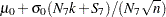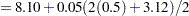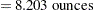where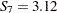is the upper sum at Hour=7, and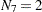is the number of successive positive upper sums at Hour=7.Previous Page | Next Page | Top of Page